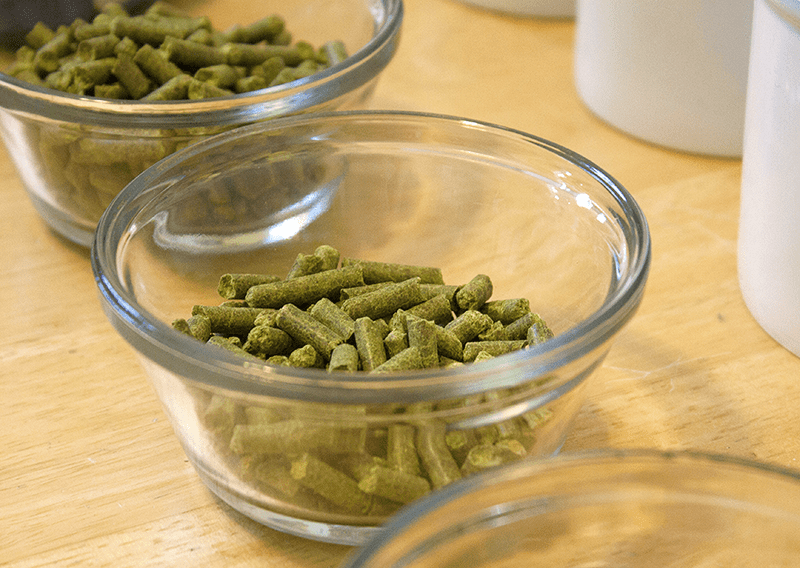If you are brewing a specific style of beer or recreating one of your previous batches, calculating your IBUs, or International Bittering Units, that are provided by the hops is one way to dial in the accuracy of your homebrew. There are plenty of free online calculators that will do this for you, but by understanding how to calculate IBUs, you can take your brewing skills to the next level. Note: IBUs only measure the hop bitterness, or isomerized alpha acids in a beer. Aromas are derived from hops oils, not alpha acids. How To Calculate IBUs You can use this calculation before your brew using estimated values, or afterwards to get a more accurate figure. Before we can calculate the IBUs of a beer, these are the factors we need to know:
• W - The weight of the hops in the homebrew recipe (usually in ounces in the US).
• AA - The alpha-acids of the hops in your recipe, expressed as AA%.
• U - Hops utilization rates (see chart at the bottom). You will need to know the length of time (in minutes) that each of the hops will be boiled in to the wort, as well as the gravity of wort at the end of the boil (in gallons).
• V - Volume of wort (in gallons) at the end of the boil.
This is the formula for calculating IBUs: IBUs = (AA% x U x W x 7489)/V But where does the 7489 come from? This is a correction value that helps us complete the formula in US units (to compute in metric units, calculate weight in grams, volume in liters, and change the correction value to 1000).So, what you will do is calculate the IBUs for each hop addition in your homebrew recipe, then add them all together to calculate the total IBUs in a beer. As an example, this past weekend I brewed a 10 gallon batch of American Brown Ale with a starting gravity of 1.060 and the following hop schedule:
• 2 oz. East Kent Goldings hops (5.2 AA%) at 60 minutes left in boil
• 1 oz. Willamette (4.7 AA%) at :30
• 2 oz. East Kent Goldings (5.2 AA%) at :10
Let's plug in some number to see how to calculate the IBUs. Starting with the first hop addition, we input the alpha acids as a decimal. (Remember to shift the decimal two places to change it from a percentage to a decimal number.) Then, we need to look at the hops utilization rate when boiled for a 60 minute boil in 1.060 gravity wort. Looking at the chart below, that number is 0.211. The weight is 2 and the volume is 10: IBUs of first addition = (.052 x .211 x 2 x 7489)/10 = 16.43 IBUs Then we do it again for each of the next two additions: IBUs of second addition = (.047 x .162 x 1 x 7489)/10 = 5.7 IBUs IBUs of third addition = (.052 x .076 x 2 x 7489)/10 = 5.92 IBUs Add them all together, and the total IBUs for this beer is about 28 IBUs.That's how to calculate IBUs. As with many things in homebrewing, IBUs are an approximation. There are a lot of factors in play, but knowing how to calculate IBUs gives you a lot more control over your brew. Plus, if you want to recreate a beer recipe that you’ve brewed before, but with hops that have different alpha acid percentages, you can use the formula to adjust the weight of each hops addition needed to arrive at the same IBUs. Do you calculate the IBUs of your homebrew? Do you use an online calculator or do you go the old fashioned route? Hops Utilization Chart----- Ed Kraus is a 3rd generation home brewer/winemaker and has been an owner of E. C. Kraus since 1999. He has been helping individuals make better wine and beer for over 25 years.

Featured products

Save \$10.00

\$77.00 \$87.00
2 reviews
Only 1 unit left
Save \$10.00

\$77.00 \$87.00
2 reviews
Only 1 unit left